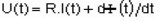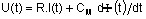# Multiplying coefficient for computation of flux through coils

## Definition

A coefficient CM (named multiplying coefficient for computation of flux through coils) is introduced for the computation of flux through coils when they are described in a finite element domain bounded by symmetries and/or periodicities.

## Concept of coil in Flux

Generally, a coil is a set of series-connected turns (stranded coil conductor).

To define completely a coil in Flux (its geometrical and electrical aspects), we must define two objects:

• an electrical component of stranded coil conductor type (the physical entity to define the value of the current)
• a coil (the geometric entity to define the shape of coils)

## Problem

What happens when a coil is described in a finite element domain bounded by symmetries and/or periodicities?

• from an electric point of view, there is only one electrical component
• from a geometric point of view, there is an "original" coil (the one described in the FE domain) and its "duplicates" by symmetry and/or periodicity

In this situation, the electrical component is a component that comprises several geometric coils.

To compute the magnetic flux through this component (i.e. through the "original" coil and its "duplicates"), Flux evaluates:

• the coefficient CM, which takes into account the number and types of symmetries and/or periodicities
• the field Conductors in series or in parallel, which takes into account the configuration type of associated conductors (all in series, all in parallel).

## Using the coefficient CM

The coefficient CM is used:

• within results post-processing to Compute flux through a coil conductor (in 3D)

(command sequence: Advanced > Predefined computation > Magnetic flux > Compute flux through a coil conductor )

• within circuit coupling

## Computation of flux through a coil conductor (postprocessor)

In general, global quantities computed in Flux (post-processing quantities) are calculated for the part of the device modeled in the finite elements domain*.

This rule applies to all the computations, carried out in the postprocessor, except for:

• quantities related to mechanical sets (force or torque) within kinematic coupling
• power balance
• flux in coils (the matter of our interest)
Note: *The user multiplies the Flux result by a specific coefficient (dependent on the number and type of symmetry and/or periodicity) to obtain the real result corresponding to the whole device.

## Computation of flux with circuit coupling (1)

With circuit coupling a coil is represented twice:

• once in the finite elements domain: region of stranded coil conductor type (or non-meshed coil in 3D)

• once in the electric circuit: electric component of stranded coil type

Reminder:

The relationship between current I, tension U and flux Φ in a conductor is written:(1)

The voltage U at the terminals of the electric component is related to flux Φ through the coil by equation 1.

If symmetries and/or periodicities are presented, the computation of flux Φ is carried out for the part of the device modeled in the finite element domain. If the characteristics of electric circuit components are the real characteristics, it is necessary to rectify the equation (1), and introduce a coefficient CM to take symmetries and/or periodicities into account.

As a result …

The relationship between current I, tension U and flux Φ in a conductor is written:(2)

The voltage U at the terminals of the electric component is related to real flux CMΦ through the coil by equation 2.

The coefficient * CM, automatically calculated by Flux, ensures coherence between the finite element domain, having symmetries and/or periodicities, and the electric circuit.

## Computation of flux with circuit coupling (2)

Concretely, two strategies are possible to ensure coherence between the finite elements domain, having symmetries and/or periodicities, and the electric circuit. These two strategies are presented below.

Strategy 1 (general case): coherence is managed by Flux

The characteristics of the electric circuit components are the real characteristics (real values of passive components (R, L, C), real values of sources...).

The coefficient CM, automatically computed by Flux, ensures coherence between the finite element domain and the electric circuit.

Strategy 2 (specific case *): coherence is the responsibility of the user

The characteristics of the electric circuit components are adjusted to take symmetries and/or periodicities into account.

The coefficient CM is set to 1 by the user.

Note: * case of linear motors

## Provided options

In the majority of cases, the computation of the coefficient CM is carried out by Flux (default option: automatic coefficient).

However, if you must impose this coefficient, provided options are detailed in the table below.

Option Description
Automatic coefficient (symmetry and periodicity taken into account) CM is automatically computed with taking active symmetries and active rotation periodicities of the problem into account.
Imposed coefficient (integer)

CM is an integer:

CM = N

N is the number of repetitive patterns described in the finite elements domain

(The finite elements domain corresponds to 1/N fraction of the real device)

Imposed coefficient (fraction)

CM is a quotient of 2 integers:

CM = N1 /N2

The finite elements domain corresponds to 1/N1 fraction of the real device.

N1 is the number of repetitive patterns described in the finite elements domain

N2 is the number of repetitive patterns supplied by the electric circuit